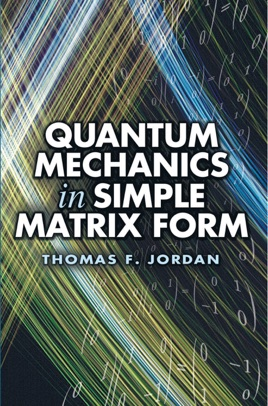• USD 12.99

## Descripción de editorial

This elementary text introduces basic quantum mechanics to undergraduates with no background in mathematics beyond algebra. Containing more than 100 problems, it provides an easy way to learn part of the quantum language and apply it to problems.
Emphasizing the matrices representing physical quantities, it describes states simply by mean values of physical quantities or by probabilities for possible values. This approach requires using the algebra of matrices and complex numbers together with probabilities and mean values, a technique introduced at the outset and used repeatedly. Students discover the essential simplicity of quantum mechanics by focusing on basics and working only with key elements of the mathematical structure--an original point of view that offers a refreshing alternative for students new to quantum mechanics.